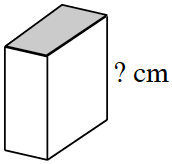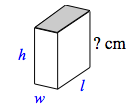### Home > CC1MN > Chapter 11 > Lesson 11.2.4 > Problem11-77

11-77.

The shaded surface of the figure at right has an area of $18$ square cm. The volume of the rectangular prism is $131.4$ cubic cm.

1. What is the height? Explain how you know.

Volume = (area of base)(height)

Rearrange this equation to solve for height.1. If the dimensions of the shaded rectangle have a ratio of $2:1$, find the surface area of the figure. Show your work.

Write an equation using the given information to solve for all the unknown dimensions of the prism. If the length is twice the width (from the ratio), then $l = 2w$, so use this in the equation for the area of the shaded side.$(\text{w})(2\text{w}) = 18 \text{ cm}^{2}$

Remember that surface area is the sum of the areas of the individual faces.
Use the dimensions you solved for above to calculate the surface area of the prism.

Surface Area = $167.4 \text{ cm}^{2}$